## 4.9.1 Self-Consistent Iteration Scheme

Figure 4.15 depicts the block diagram of the iterative procedure employed to solve the coupled system of transport and POISSON equations. All the procedures are discussed in the following.

We solve the kinetic equations in mode-space, (4.25) and (4.26), to obtain the GREEN's functions. The required elements for calculating the GREEN's functions are the HAMILTON ian, and the electron-phonon and contact self-energies. As discussed in Section 4.4, diagonal elements of the HAMILTONian are potential energies, which can be obtained from the solution of the POISSON equation, and off-diagonal elements represent the coupling between adjacent rings of carbon atoms in the CNT. Given the contact properties and the contact-device coupling parameters, the contact self-energy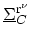can be calculated once at the start of the simulation (see Section 4.5).

The calculation of the electron-phonon self-energy is presented in Section 4.6. Within the self-consistent BORN approximation of the electron-phonon self-energy (Section 3.6), the non-interacting GREEN's function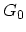is replaced by the full GREEN's function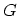. However, the full GREEN's is the not known and has to be calculated. As a result, a coupled system of equations is achieved which can be solved by iteration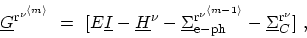(4.61)

wheredenotes the iteration number. For the first step the electron-phonon self-energy is assumed to be zero and the GREEN's function is calculated from the kinetic equation. The next iteration starts with the calculation of the electron-phonon self-energy based on the GREEN's function from the previous iteration. The updated electron-phonon self-energy is then used for the calculation of the GREEN's function. This iteration continues till a convergence criterion is satisfied. The mentioned procedure should be followed for each subband (mode) and finally the total charge density is calculated.

In semi-classical simulations, the coupled system of the transport and POISSON equations is solved by GUMMEL's or NEWTON's method . Both GUMMEL's method  and a variation of NEWTON's method  can be employed in self-consistent quantum mechanical simulations. While GUMMEL's method has a fast initial error reduction, for NEWTON's method it is very important that the initial guess is close to the solution. The computational cost per iteration of NEWTON's method can be much higher than that for GUMMEL's method.

We employed GUMMEL's method, where after convergence of the electron-phonon self-energy the POISSON equation is solved once. Based on the updated electrostatic potential, the GREEN's functions and the electron-phonon self-energy are iterated again. These two iterations continue until a convergence criterion is satisfied. Finally, the total current through the device is calculated.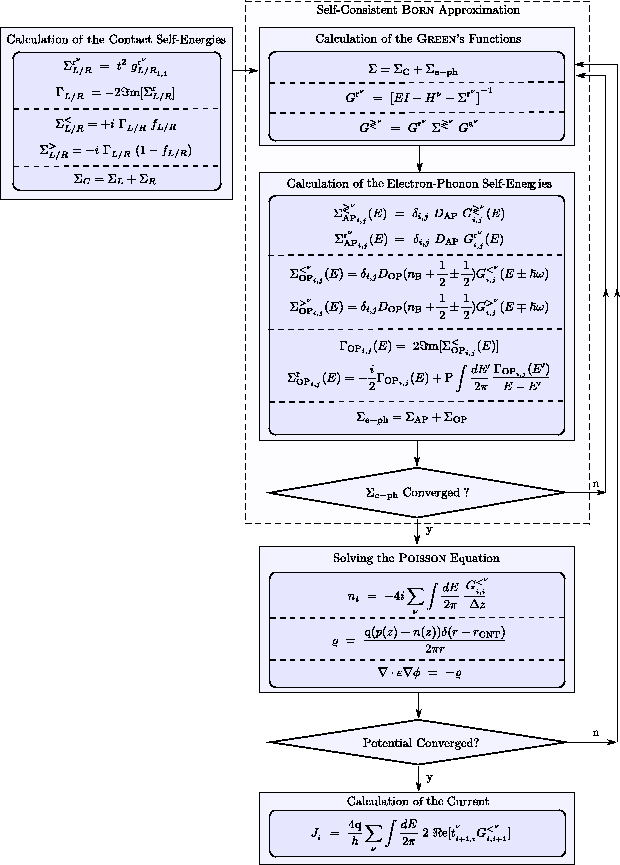M. Pourfath: Numerical Study of Quantum Transport in Carbon Nanotube-Based Transistors